# Base Excitation Absolute Result?

Member Posts: 7

I am solving transient analysis by applying base excitation to a structure there is a function in the acceleration setup that appear when I set it to base excitation called absolute result. This function if I set it to "No" the supports get fixed and the body deform about and the stresses are low as well as the deformation. when I set it to "Yes" the supports start to move from their places and showing very high stresses about 7300 MPa. I checked a guide for what is meant by absolute result, this is what I found (link at end of the post):
"The Absolute Result property is set to Yes by default. As needed, change the value to No if you do not want to include enforced motion."
I want to understand what is meant by absolute result (enforced motion), and why the stresses are so excessive, I supposed to simulate a load coming from a bump that is damped from tires and suspensions then applied to my chassis at the support point. The acceleration object load is resultant of the suspension (tabular data).

https://www.sharcnet.ca/Software/Ansys/17.0/en-us/help/wb_sim/ds_Acceleration.html

• edited December 2019

Was the acceleration load measured by an accelerometer on the structure of a real vehicle going over a bump?  Where on the structure was the accelerometer? You say you are using that data to load the frame.  How are you supporting the frame?  Do you have all the mass of the vehicle attached to the frame? Please reply and insert images of your structure. Indicate which faces are Fixed Supports and if you have a non-zero Enforced Displacement, indicate that face too.

Below is a simple example that explains the difference between Absolute = No and Absolute = Yes.

The frame is represented by a rectangular plate.  It is fixed at end A, which represents where the wheel has an input to the frame. At the other end B is a point mass which represents all the mass of the vehicle excluding the rectangular frame.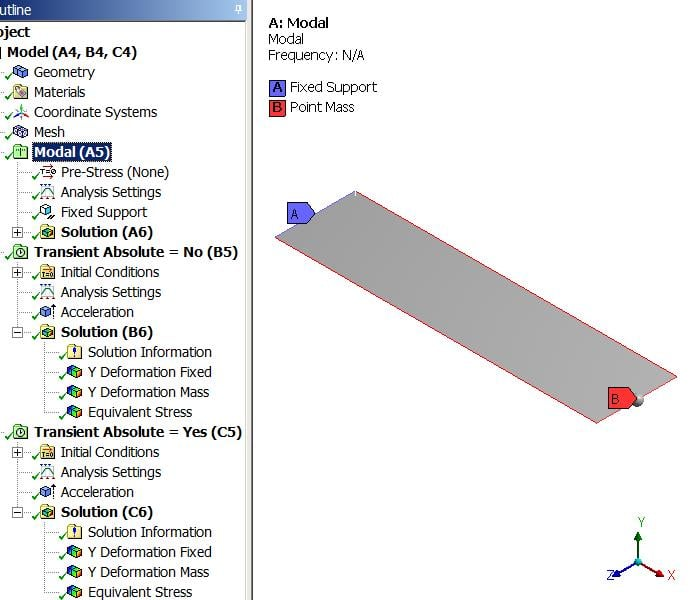A modal analysis is linked into two Transient Structural analyses to look at the effect of changing Absolute results from No to Yes.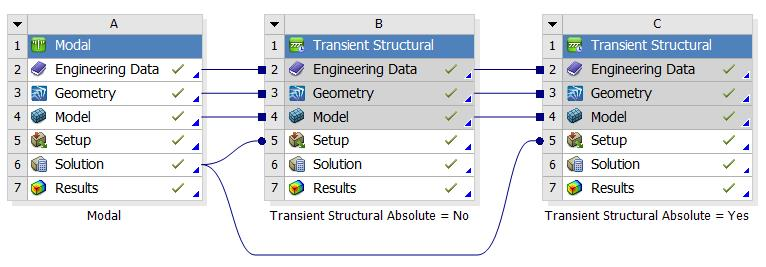The acceleration load in the two Transients is a Base Acceleration in the Y direction on the Fixed Support.
The graph below is in the units of m/s^2.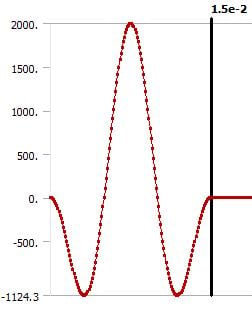There are two Directional Displacement plots. One on the Fixed Support A end and the other on the Mass B end.

## Absolute = Yes analysis

The graph of the displacement of the Fixed Support A end shows the frame being forced down 11 mm and pulled back up over a 15 ms period, then held at zero for the remainder of the 100 ms duration. This is the acceleration input being integrated twice to get to displacement.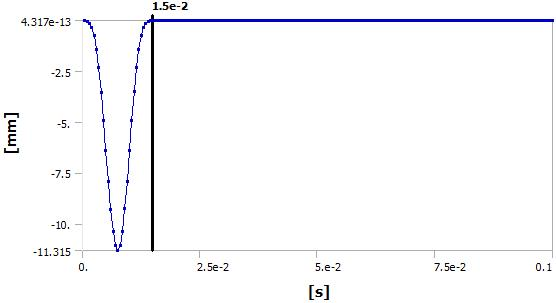Here is the response of the Mass at the B end. While the other end is forced rapidly down and up, the flexibility of the frame and the substantial mass at the other end allows it to respond more gradually to the disturbance at the "fixed" end.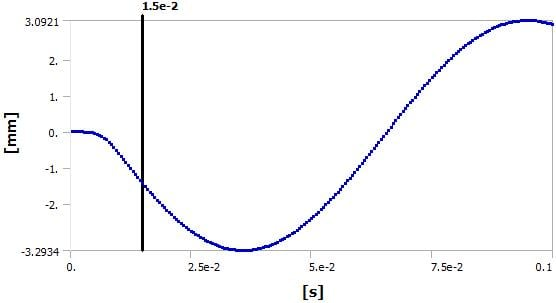Both the above plots are relative to ground when the Base is moving according to the acceleration input.

## Absolute = No analysis

The graph of the displacement of the Fixed Support A end shows zero displacement.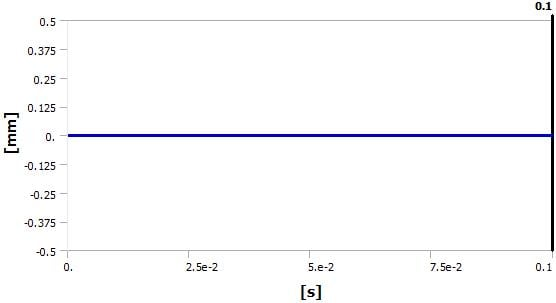Here is the response of the Mass at the B end. Relative to the fixed end, the mass goes up 11 mm and down over 15 ms then begins to oscillate.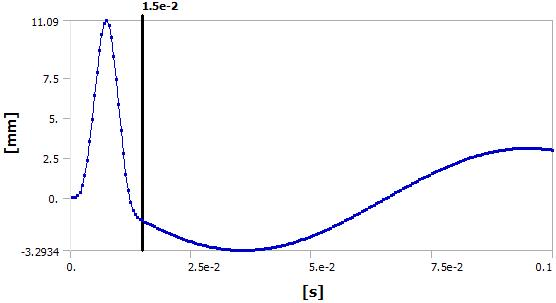These graphs are not relative to absolute ground, but measured from the point of view of a ruler on the "fixed" end.

## Stress Results from the two analyses

The stress results from the two analyses are identical, because all the Absolute Result Yes/No choice is doing in this model is changing the point of view of the deformations, which has no effect on the stress.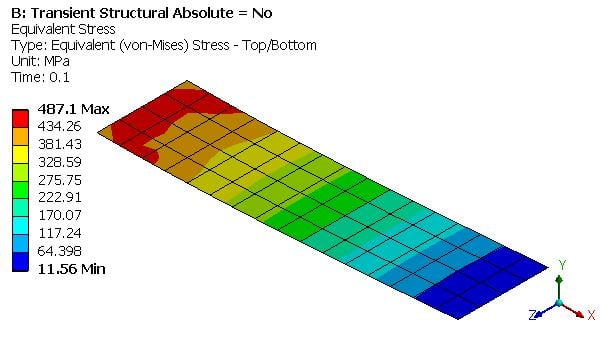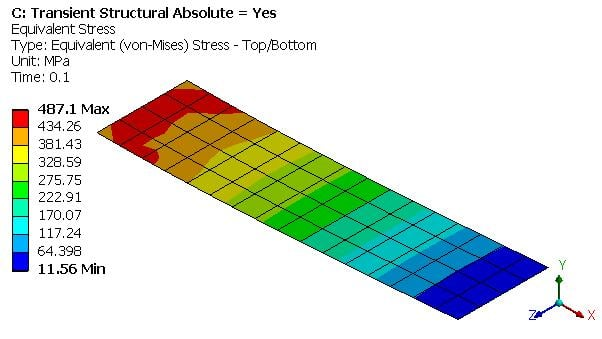I tried to set a base excitation in a transient analysis without running a prior modal analysis and found it is not possible. why is that?

And how does the modal analysis effect the transient analysis. Until now I was thinking there is no relation between these two, in contrast to the case of using modal analysis before harmonic analysis or eigenvalue buckling..

• Base excitation is only available in Modal Superposition (MSUP) Transient Structural models. In the Modal, the base was required to be Fixed. No non-zero displacements are allowed in Modal. Therefore, Base Excitation is a work around to get a MSUP Transient to have a Base Excitation.

If you have a Full Transient Structural, with no linked Modal, then you just apply the acceleration load to the base as an acceleration load. It is a base excitation, but done directly, there is no need for a work around.

When the model is linear, you spend less time waiting for a solution by doing a MSUP Transient because it takes some time to extract the modes but then it is very fast to integrate those few equations over the simulation time.

A Full Transient Structural has a huge number of equations to integrate over the simulation time, so that is very slow, but it your model is nonlinear, you have no choice.

• Thanks.

If I don't miss something, acceleration can be applied to the whole body only - there is no way to constrain just the acceleration of one face. In this case, when running a Full Transient Structural, I can fix one face and apply alternating acceleration field. While in this simple case both approaches seem to be identical (fixed face, changing acceleration field vs accelerating face) - I'm not sure they will end up with the same results for more complicated scenarios.

Is there a way to define the acceleration of a face in a transient simulation?

• edited September 2020

Inertial loads (acceleration, gravity, rotational velocity) act on all the mass in the model.

When you pick a face, you can apply a Force, Moment or Pressure loads, or you can apply Displacement or Velocity boundary conditions.

If you integrate transient acceleration data, you get velocity, so you could apply it that way.

• In case if the applied acceleration values are absolute (Earthquake time history) then what about the response values ? The response deformations and acceleration are also absolute or will be relative if I am applying the acceleration at the base of the structure with absolute option on?

• In a Full Transient, everything is absolute.

If you want relative, you have to subtract the base input manually.